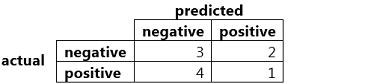# The formula for recall is given by (True Positives) / (True Positives + False Negatives).

The formula for recall is given by (True Positives) / (True Positives + False Negatives).

What is the recall for this example?A. 0.2

B. 0.25

C. 0.5

D. 0.33

Reference: https://machinelearningmastery.com/precision-recall-and-f-measure-for-imbalanced-classification/